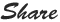# PHP While Loop

`````` PHP While Loop:
While loop starts and ends with opening and closing backers respectively, while condition needs to be declared on first position.
<body>
<?php
\$y = 2;
while(\$y <= 21) {
echo "This is table of two : \$y <br>";
\$y= \$y+2;
}
?>
</body>
Output:
This is table of two : 2
This is table of two : 4
This is table of two : 6
This is table of two : 8
This is table of two : 10
This is table of two : 12
This is table of two : 14
This is table of two : 16
This is table of two : 18
This is table of two : 20
``````